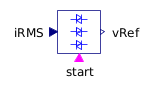Single Phase Winding - MapleSim Help

Fundamental Wave Single Phase Winding

Symmetric winding model coupling electrical and magnetic domainDescription The Fundamental Wave Single Phase Winding (or Single Phase Winding) component models a single-phase winding. It consists of a single-phase electromagnetic coupling, a resistor in series with the electrical ports, and a symmetrical stray inductor modeled by a reluctance across the magnetic ports. An optional heat port allows thermal flow from the resistor.Connections

 Name Description Modelica ID ${\mathrm{pin}}_{p}$ Positive pin pin_p ${\mathrm{pin}}_{n}$ Negative pin pin_n ${\mathrm{port}}_{n}$ Negative complex magnetic port port_n ${\mathrm{port}}_{p}$ Positive complex magnetic port port_p Heat Port Winding Heat port of winding resistor heatPortWindingParameters

 Name Default Units Description Modelica ID $\mathrm{\alpha }$ $0$ $\frac{1}{K}$ Temperature coefficient of winding at 20 degC alpha20 ${L}_{\mathrm{\sigma }}$ $H$ Winding stray inductance per phase Lsigma ${n}_{\mathrm{eff}}$ $1$ $1$ Effective number of turns per phase effectiveTurns ${R}_{\mathrm{ref}}$ $\mathrm{\Omega }$ Winding resistance per phase at TRef RRef ${T}_{\mathrm{oper}}$ $293.15$ $K$ Operational temperature of winding TOperational ${T}_{\mathrm{ref}}$ $293.15$ $K$ Reference temperature of winding TRef $\mathrm{\theta }$ $\mathrm{rad}$ Orientation of the resulting fundamental wave field phasor orientation Use Heat Port $\mathrm{false}$ True (checked) means heat port is enabled useHeatPortModelica Standard Library The component described in this topic is from the Modelica Standard Library. To view the original documentation, which includes author and copyright information, click here.